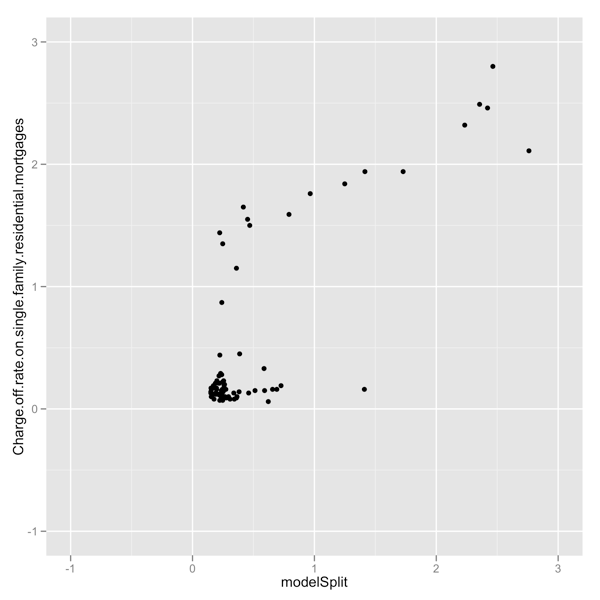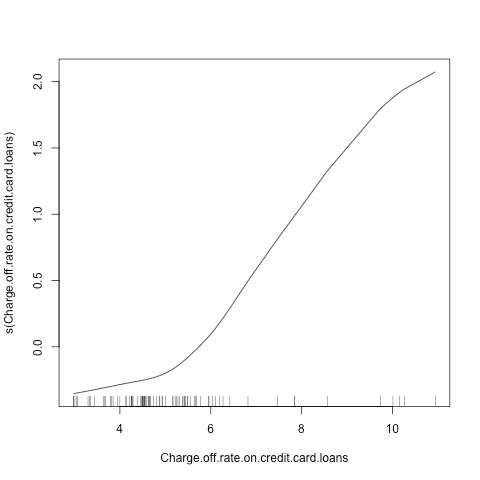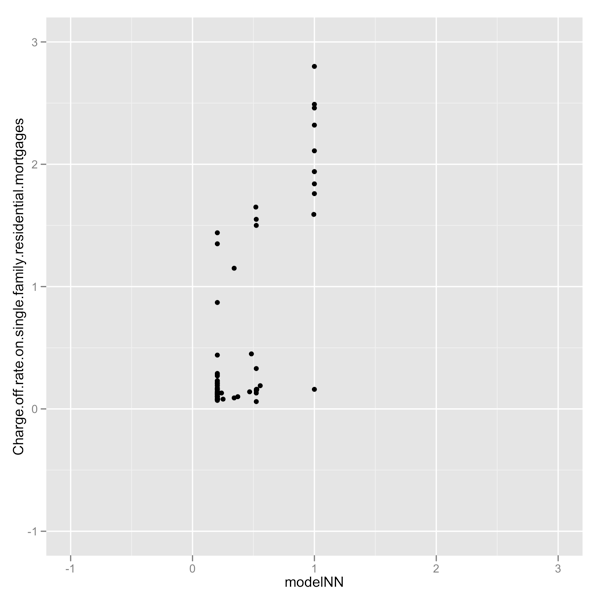A primary problem data scientists face again and again is: how to properly adapt or treat variables so they are best possible components of a regression. Some analysts at this point delegate control to a shape choosing system like neural nets. I feel such a choice gives up far too much statistical rigor, transparency and control without real benefit in exchange. There are other, better, ways to solve the reshaping problem. A good rigorous way to treat variables are to try to find stabilizing transforms, introduce splines (parametric or non-parametric) or use generalized additive models. A practical or pragmatic approach we advise to get some of the piecewise reshaping power of splines or generalized additive models is: a modeling trick we call “masked variables.” This article works a quick example using masked variables.To start let us get a dataset derived from Federal Reserve Data. To prepare we downloaded and prepared a consumer default rate dataset. We give below R code to download the data set and attempt to model single family residential mortgage charge-off (or loss) rates as a linear function of credit chard charge off rates.

``` library(ggplot2) d <- read.table('https://github.com/WinVector/Examples/tree/main/dfiles/maskVars/FRB_CHGDEL.csv', sep=',',header=T) model1 <- lm(Charge.off.rate.on.single.family.residential.mortgages ~ Charge.off.rate.on.credit.card.loans,data=d) d\$model1 <- predict(model1,newdata=d) summary(model1) plot1 <- ggplot(d) + geom_point(aes(x=model1, y=Charge.off.rate.on.single.family.residential.mortgages)) + xlim(-1,3) + ylim(-1,3) #ggsave('plot1.png',plot1) cor(d\$model1,d\$Charge.off.rate.on.single.family.residential.mortgages, use='complete.obs') # 0.7706394 ```

The plot below shows the performance of this trivial model (which ignores auto-correlation, inventory, dates, regional factors, macro-econmic factors and regulations). What we see is the model incorrectly predicts continuous variation between zero and one percent when actual mortgage charge-offs are more of a step function (the rate stays near zero until it jumps above one percent). Even so the correlation of this model to actuals is 0.77, which is fair.Any one variable linear model is really just a shift and rescaling (or an affine transform) of the single input variable. So we get the exact same shape and correlation if we skip the linear modeling step and directly plot the relation between the two variables. We show this in the R code and graph below.

``` plotXY <- ggplot(d) + geom_point(aes(x=Charge.off.rate.on.credit.card.loans, y=Charge.off.rate.on.single.family.residential.mortgages)) ggsave('plotXY.png',plotXY) cor(d\$Charge.off.rate.on.credit.card.loans, d\$Charge.off.rate.on.single.family.residential.mortgages, use='complete.obs') # 0.7706394 ```Now we get to the meat of the masked variable technique. We want to build a step-wise function that better fits the relation. To do this the analyst either by hand or through automation could note in our last graph residential mortgages charge-off rates do not seem to be very sensitive to credit card charge off rates until the credit card charge-off rate exceeds 5%.

To encode this domain knowledge we build three new synthetic variables: an indicator that tells us if the credit card charge-off rate is over 5% or not. We call this variable HL (high/low indicator). We then multiply this new variable by our original variable to get a new variable that only varies when the charge-off rate is above 5% (we call this variable H and it is an interaction between the new indicator variable and the original variable). Finally we create a third variable that varies only when the credit card charge-off rate is no more than 5%. This variable is equal to (1-HL) times the original variable and we call it L. We call HL the mask and H and L masked variables. The R-code to form these three new synthetic variables is given below:

``` d\$Charge.off.rate.on.credit.card.loans.HL <- ifelse(d\$Charge.off.rate.on.credit.card.loans > 5,1,0) d\$Charge.off.rate.on.credit.card.loans.H <- with(d,Charge.off.rate.on.credit.card.loans.HL*Charge.off.rate.on.credit.card.loans) d\$Charge.off.rate.on.credit.card.loans.L <- with(d,(1-Charge.off.rate.on.credit.card.loans.HL)*Charge.off.rate.on.credit.card.loans) ```

We can now use these new variables to build a slightly better model. We do this by exposing all three synthetic variables to the fitter. Thus the fitter now has available in its concept space all step-wise linear functions with a change at 5% (including discontinuous functions). This is related to kernel tricks: make the unknown function you want a linear combination of functions you have and a standard linear fitter can find it for you. The R-code and graph are given below:

``` modelSplit <- lm(Charge.off.rate.on.single.family.residential.mortgages ~ Charge.off.rate.on.credit.card.loans.HL + Charge.off.rate.on.credit.card.loans.H + Charge.off.rate.on.credit.card.loans.L,data=d) d\$modelSplit <- predict(modelSplit,newdata=d) summary(modelSplit) plotSplit <- ggplot(d) + geom_point(aes(x=modelSplit, y=Charge.off.rate.on.single.family.residential.mortgages)) + xlim(-1,3) + ylim(-1,3) #ggsave('plotSplit.png',plotSplit) cor(d\$modelSplit,d\$Charge.off.rate.on.single.family.residential.mortgages, use='complete.obs') # 0.8133998 ```Notice we now get a better correlation of 0.81 and the graph shows that the model is more accurate in the sense its predictions are also clustered near zero (without the horizontal stripe that represented mis-predicted variation).

Now we could call this modeling technique a “poor man’s GAM.” What a GAM does is try to learn the optimal re-shaping of a variable for a given modeling problem. That is instead of the analyst picking a cut-point and asking the modeling system to find slopes (which is what we did when we introduced separate masked variables) we ask the modeling system to learn a best re-shaping. The R-code and graph for a GAM fit are given below. Notice the `s()` wrapper which tells the GAM to think about reshaping a given variable.

``` library(gam) modelGAM <- gam(Charge.off.rate.on.single.family.residential.mortgages ~ s(Charge.off.rate.on.credit.card.loans),data=d) summary(modelGAM) d\$modelGAM <- predict(modelGAM,newdata=d) plotGAM <- ggplot(d) + geom_point(aes(x=modelGAM,y=Charge.off.rate.on.single.family.residential.mortgages)) + xlim(-1,3) + ylim(-1,3) #ggsave('plotGAM.png',plotGAM) #png(filename='gamShape.png') plot(modelGAM) #dev.off() cor(d\$modelGAM,d\$Charge.off.rate.on.single.family.residential.mortgages,use='complete.obs') # 0.8160738 ```

The GAM correlation of 0.82 is slightly better than our masked model. And we can ask the GAM to show us how it reshaped the input variable. Notice the shape the GAM splines picked is a hockey stick (piece wise linear continuous curve) with the bend near 5%.``` #png(filename='gamShape.png') plot(modelGAM) #dev.off() cor(d\$modelGAM,d\$Charge.off.rate.on.single.family.residential.mortgages, use='complete.obs') # 0.8160738 ```For completeness we include a neural net fit, but we haven’t tuned its controls or hyper-parameters so it is a fully fair comparison. We just want to emphasize the properly using a neural net takes some work (isn’t completely free). And we feel if you are going to work on variables you are better off using techniques like variable transforms, treatments or masks.

``` library(nnet) modelNN <- nnet(Charge.off.rate.on.single.family.residential.mortgages ~ Charge.off.rate.on.credit.card.loans,data=d, size=3) d\$modelNN <- predict(modelNN,newdata=d) plotNN <- ggplot(d) + geom_point(aes(x=modelNN, y=Charge.off.rate.on.single.family.residential.mortgages)) + xlim(-1,3) + ylim(-1,3) #ggsave('plotNN.png',plotNN) cor(d\$modelNN,d\$Charge.off.rate.on.single.family.residential.mortgages, use='complete.obs') # 0.7961966 ```The point of the masked variable technique is: it represents a good compromise between using analyst/data-scientist reasoning and sophisticated packages. The masking cuts can be generated once by an analyst and supported by providing the documenting graphs as we have shown here. Then an already in-place standard fitting system can pick the coefficients for the new synthetic variables (causing the fitter itself to compute the shape of the optimal piece-wise curve, saving the analyst this chore). This technique can be used in any data analysis environment that supports graphing, user-defined transformations and regression fitting (linear or otherwise).

The technique doesn’t require the analyst to pick the actual transform or slopes (again, the fitter does this). Also, this methodology is good for supporting audit and maintenance. The construction of synthetic variables can be documented and validated and standard explainable methods can be used for the remainder of the fitting process. We feel the masked variable trick represents a good practical compromise in terms of power, rigor and clarity.### jmount

Data Scientist and trainer at Win Vector LLC. One of the authors of Practical Data Science with R.